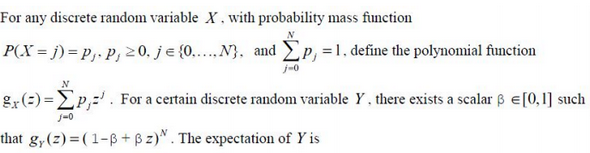# GATE | GATE-CS-2017 (Set 2) | Question 59(A) Nβ(1-β)
(B)
(C) N((1-β))
(D) Not expressible in terms of N and β

Explanation: gy(z) = ((1- β)βz)N

Expanding  gy(z) we’ll get binomial distribution  with n=N and p = β

Mean of binomial distribution is E[Y] = n*p = Nβ which seems to be the only correct option here.

Therefore, option B

Quiz of this Question

My Personal Notes arrow_drop_up
Article Tags :

Be the First to upvote.

Please write to us at contribute@geeksforgeeks.org to report any issue with the above content.